U.S. Dept. of Commerce / NOAA / OAR / PMEL / Publications

## Variability of the sea surface temperature in the eastern equatorial Pacific during 1986-88

### (Mixed Layer Temperature Variability, continued)

#### Surface Heat Flux and Penetrative Radiation

The terms in the surface heat flux into the mixed layer can be estimated using the bulk formulae. These relations are discussed in several recent references [e.g., McPhaden, 1982; Stevenson and Niiler, 1983; Reed, 1983; Dobson and Smith, 1988; Weare, 1989]. The equations used here are those of Reed ; their accuracy is evaluated in Dobson and Smith . Specifically, the surface heat flux terms in (1) are given by: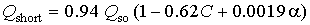(2)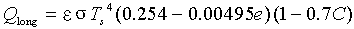(3)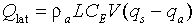(4)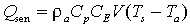(5)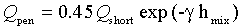(6)

In (2), Q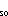is the clear sky radiance [Reed, 1977, 1983], C the cloudiness (tenths) and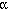is the noon solar altitude (degree). The clear sky radiance and alpha are evaluated using a harmonic formula which introduces latitudinal and annual variations. In (3),= 0.97 is the emissivity of the ocean;= 5.67 × 10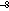W m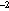°K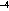is the Stefan-Boltzmann constant, e is the atmospheric vapor pressure. In (4) and (5),= 1.15 × 10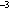g cmis the air density, C= 1.2 × 10is the exchange coefficient, L = 2440 J g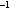is the latent heat of vaporization, V is the wind speed at a nominal height of 10 m, qis the specific humidity saturated at the sea temperature, qis the specific humidity of the air at 10 m elevation, and Tand Tare the air and sea temperatures. Equation (6) estimates the heat loss by radiation which penetrates through the mixed layer. This exponential decay law is taken from Ivanov  with= 0.04 m. The mixed layer depth, h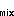, is estimated as discussed below.

The mooring measurements provided information on wind speed, SST and air temperature. In order to apply equations (2-5), we used the mooring data supplemented by Comprehensive Ocean-Atmosphere Data Set (COADS) marine surface climatology. Measured winds and air temperature were used without correcting the measurement height to 10 m. Assuming a logarithmic profile with neutral stability and a constant drag coefficient of 1.2 × 10[Large and Pond, 1982], an increase of wind speed by 8% would account for the measurement height. This correction was not made because of the uncertainties in the assumptions used to derive it. The saturation humidity qin (4) was evaluated from the Clausius-Clapeyron equation using the observed SST. Cloud cover and relative humidity were obtained from a monthly mean COADS climatology for 1946-85 [Deser, 1989]. For comparison, monthly mean values based on COADS data for 1986-1988 were also used (C. Deser, personal communication, 1989). Liu and Niiler  discuss the sensitivity of the latent heat flux to variations in humidity.

After smoothing with a 91-day Hanning filter, the estimated surface flux terms are shown in Figure 4. The incoming shortwave radiation is based solely on climatology. It has a semiannual variation associated with the sun passing over the equator twice each year. No interannual variability is possible because climatological monthly cloud cover is used in (2). The mean value is about 250 W mand the standard deviation of the low pass filtered time series is about 15 W m. These results are consistent with Reed .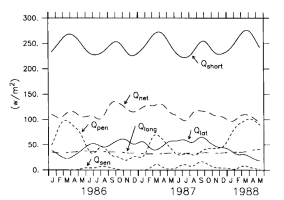Fig. 4. Components of the surface heat flux (see equation (1)).

The estimated latent heat flux undergoes both annual and interannual variations. Latent heat flux is minimum in March, when wind speed is low (Figure 3), and maximum in boreal autumn. The minimum in March of 1987 is almost twice the March value in 1986 or 1988. This increase in latent heat release is related to the warm SST anomalies and higher wind speeds that occurred in the eastern Pacific during the 1987 El Nińo. The mean latent heat flux is approximately 45 W m, which again agrees with Reed's result, and the standard deviation is about 12 W m.

The longwave radiation is almost a constant value of 35 W mover the record length and thus does not contribute significantly to the change in the total heat flux. The sensible heat flux depends on the air-sea temperature difference. Previous studies in the Pacific Ocean [Reed, 1977, 1983; Weare et al., 1981; Esbensen and Kushnir, 1981] have shown that this heat flux is small (about 10 W m). Since some of the air temperature data are missing from the equatorial mooring record, we were unable to compute the sensible heat flux over the entire record length. However, whenever possible the calculation was made and the mean (and standard deviation) of Q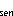was less than 5 W m. Thus Qwas ignored in subsequent calculations.

Because mixed layer depth is shallow in the eastern Pacific, incoming solar radiation cannot be completely absorbed within the layer. On average, about 50 W mpenetrates into the deep ocean. This penetrative radiation is strongest during springtime when the mixed layer is shallow (Figure 5). Thus although the solar radiation is strongest in spring season, the net heating received by the mixed layer is not necessarily largest at that time. Indeed, the net heating within the mixed layer, Q, in Figure 4, is maximum in the fall of 1986. The penetration of radiation through the mixed layer reduces the annual variation of the net solar heating. The mean value of the net heating within the mixed layer is 115 W mwith a standard deviation of 10 W m. The mean heat flux at the surface is 165 W m, comparable to the 150 W mvalue determined by Reed  for the eastern equatorial Pacific.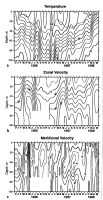Fig. 5. Contour plots of (a) isotherm depth (contours are in degrees Celsius), (b) zonal velocity (centimeters per second), and (c) meridional velocity (centimeters per second) in the upper 100 m at 0°, 110°W, based on the 91-day low-pass-filtered time series. The dashed line superimposed on the isotherm contours indicates the mixed layer depth computed as discussed in the text. Contour intervals are 1°C, 20 cm s, and 5 cm sfor temperature, zonal velocity, and meridional velocity, respectively.

Sources of errors in Qcan come from both random and systematic uncertainties in the basic observations and in the parameters in the bulk formulae. Recent studies by Weare  indicate that the uncertainties in monthly mean climatological estimates of the surface heat flux components over the eastern tropical Pacific are 20 W mfor the solar radiation, 10 W mfor longwave radiation, 35 W mfor latent heat flux, and 5 W mfor sensible heat flux, which leads to a total error of 40 W mfor the net surface heat flux. The uncertainties of the variability of heat fluxes should be smaller than the error in absolute flux because systematic errors in the observations and bulk formulae may cancel upon differencing. However, our estimates have an additional uncertainty associated with the use of climatological data combined with mooring data for specific years. Comparison of the fluxes computed from climatological values of cloud cover and relative humidity (Figure 4) with those estimated using 1986-1988 COADS data show an rms difference of about 10 W m. Thus, the deviations from climatology of cloud cover and humidity during the study period were relatively small. The climatological values were used in the remaining calculations since the statistical reliability of these estimates is higher.

Go back to previous section or forward to next section

PMEL Outstanding Papers

PMEL Publications Search

PMEL Homepage